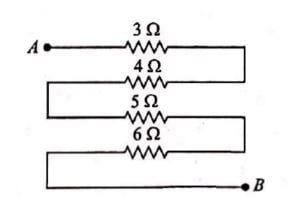Equivalent resistance (in ohm) of the given network is• a)
28
• b)
18
• c)
26
• d)
25

### Related TestEduRev NEET Jan 21, 2022Between points A and B all resistances are combined in series
∴ Req = 3Ω + 4Ω + 5Ω + 6Ω
= 18Ω
Between points A and B all resistances are combined in series
∴ Req = 3Ω + 4Ω + 5Ω + 6Ω
= 18Ω

Between points A and B all resistances are combined in series∴ Req= 3Ω + 4Ω + 5Ω + 6Ω= 18ΩBetween points A and B all resistances are combined in series∴ Req= 3Ω + 4Ω + 5Ω + 6Ω= 18Ω
Do you know? How Toppers prepare for NEET Exam
With help of the best NEET teachers & toppers, We have prepared a guide for student who are preparing for NEET : 15 Steps to clear NEET Exam